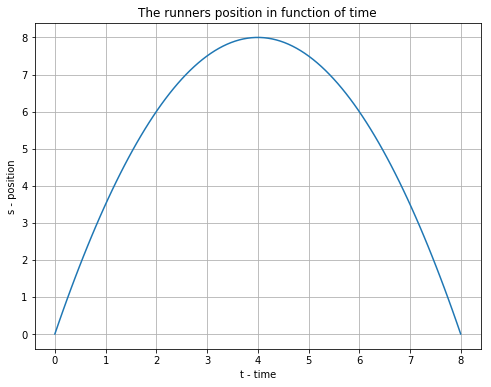Chapter-2 Q023 - Physics For Engineers And Scientists - SolutionsON7AMI Amateur Radio, Meteology and Astronomy website. JO10UX# Question 23 - Problems - Chapter 2¶

## Problem:¶

The position of a runner is given by $x = 4.0t-0.50t^2$, where x is in meters and t is in seconds.

## Question:¶

What is the average speed between t = 0 and t = 8.0 s?

(Hint: Find the maximum value of x to determine each of the outward and backward distances.)

## Solution:¶

The runner's trajectory is described by a parabola. He thus travels back and forth in distance.

The factor of t squared is negative so the top of the parabola points upwards.

We can determine the furthest distance the runner travels when the derivative of the distance is zero.

$$\frac{dx}{dt}=\frac{d}{dt}(-0.5t^2+4t)$$

We find the turn aroud point by solving for speed = 0

$$\implies-0.5x2t+4=0$$

$$\implies t=\frac{-4}{-1}$$

$$\implies t = 4$$

We could also let Python do the derivative using the Sympy (Symbolic Python) library. by mean of excersice we will do this here.

In :
from sympy import *
import numpy as np
import matplotlib.pyplot as plt
t = symbols('t')
x = diff((4*t - 0.5*(t**2)),t,1)   # solve the derivative into t
print(f'The derivative is ({x})')
s = solve(x,t) # here We solve the result in t (for v = 0 the moment of turn around)
# there is only one solution so we use element 0 of our solutions
print(f'The turn arround point is at {s:.2f} seonds')

The derivative is (-1.0*t + 4)
The turn arround point is at 4.00 seonds


The distance run after the time he reached the turn around point is:

$$s_{half} = \Big|4.(4s) - 0.5.(4s)^2\Big|$$

In :
s_half = np.abs(4*4-1.5*4**2)
Time = 8 #s
print(f'The turn around point is at {s_half:.1f} m so the total distance is {2*s_half:.1f} m')
v_mean = (2 * s_half)/Time
print(f'The average speed over 8 seconds is {v_mean:.2f} m/s')

The turn around point is at 8.0 m so the total distance is 16.0 m
The average speed over 8 seconds is 2.00 m/s

In :
t_val = np.linspace(0,8,100)
s_val = 4*t_val - 0.5*t_val**2
plt.figure(figsize=(8,6))
plt.plot(t_val, s_val)
plt.grid()
plt.title('The runners position in function of time')
plt.xlabel('t - time')
plt.ylabel('s - position')

Out:
Text(0,0.5,'s - position')سُوۡرَةُ حٰمٓ السجدة / فُصّلَت ثُمَّ اسْتَوَىٰ إِلَى السَّمَاءِ وَهِيَ دُخَانٌ فَقَالَ لَهَا وَلِلْأَرْضِ ائْتِيَا طَوْعًا أَوْ كَرْهًا قَالَتَا أَتَيْنَا طَائِعِينَ ﴿١١ فَقَضَاهُنَّ سَبْعَ سَمَاوَاتٍ فِي يَوْمَيْنِ وَأَوْحَىٰ فِي كُلِّ سَمَاءٍ أَمْرَهَا ۚ وَزَيَّنَّا السَّمَاءَ الدُّنْيَا بِمَصَابِيحَ وَحِفْظًا ۚ ذَٰلِكَ تَقْدِيرُ الْعَزِيزِ الْعَلِيمِ ﴿١٢ Surah Fussilat: Thereafter turned He to the heaven and it was as smoke, and said Unto it and Unto the earth: come ye twain, willingly or loth. They said: we come willingly. (11) Then He decreed them as seven heavens in two days, and revealed Unto each heaven the command thereof; and We bedecked the nether heaven with lamps and placed therein a guard. That is the ordinance of the Mighty, the Knower. (12) De hemelen en aarde (waren) een samenhangende massa. Wij hebben ze toen van elkaar gescheiden… Wij hebben de hemel tot een beschermend dak gemaakt … en de dag en de nacht, de zon en de maan geschapen. (Koran 41 11:12) Copyright (c) 2000-2020 - Jean Paul Mertens - ON7AMI - formal ON1AMI - Grote Steenweg 86 - 9840 Zevergem - Belgium - Europe